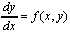# Various Calculators

## Hewlett Packard HP 38G:

Please note: The first time each program function is exercised on the HP 38G, before executing the code, the calculator first takes a modest amount of time to compile it. As a consequence, all subsequent uses of that particular program function will run immediately and also much more quickly than they otherwise would. The latter two programs given below contain several different components, each of which must be compiled separately before running the first time. This approach to code storage and use is significantly different from that employed by the HP 48G(X).

• RIEMANN Calculates Several Riemann Sums.
• RSUM Calculates and Displays the Approximating Areas for Several Types of Riemann Sums
• SLOPEFIELD Displays a Field of Slopes for.

## Hewlett Packard HP 48G(X):

• RSUM Calculates Several Riemann Sums
• RIEMANN Calculates and Displays the Approximating Areas for Several Types of Riemann Sums

• Slopefield plotting is available in a built-in plotting mode - read your manual.

## Texas Instruments TI 82 and TI 83:

• RSUM Calculates Several Types of Riemann Sums
• SLOPEFIE Displays a Field of Sample Slopes for.

## Texas Instruments TI 85 and TI 86:

• RSUM Calculates Several Types of Riemann Sums
• SLOPEFIE Displays a Field of Sample Slopes for.

## CASIO CFX-9800G-w:

• RIEMANN Calculates Several Types of Riemann Sums

You must type this program into your calculator yourself.

## Sharp Electronics EL 9300C:

• 'riemann' Calculates Several Types of Riemann Sums
• 'slopefield' Displays a Field of Sample Slopes for.